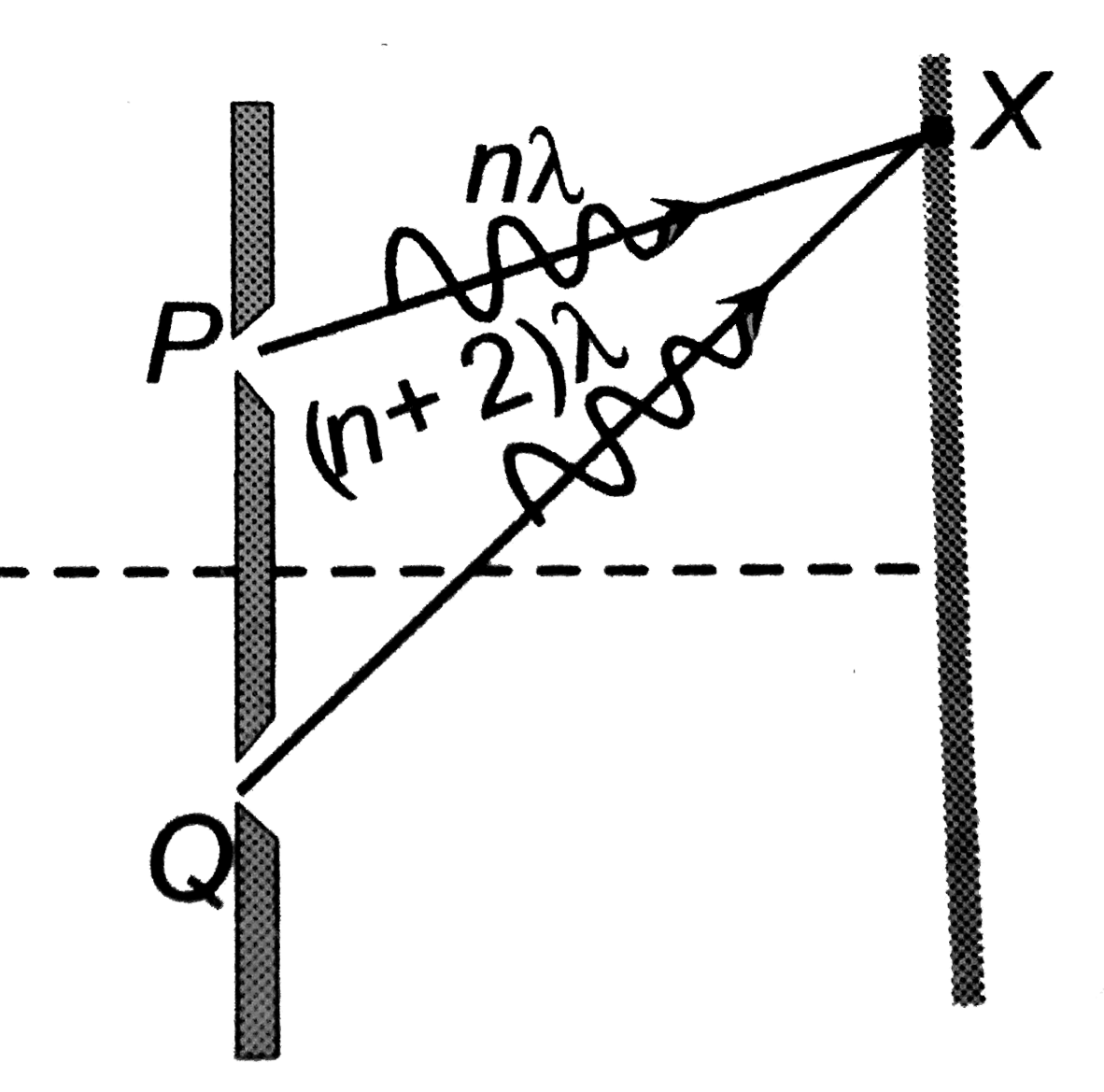# The figure shows a double slit experiment with P and Q as the slits. The path lengths PX and QX are nlambda and (n+2)lambda respectively, where n

45 views
in Physics
The figure shows a double slit experiment with P and Q as the slits. The path lengths PX and QX are nlambda and (n+2)lambda respectively, where n is a whole number and lambda is the wavelength. Taking the central fringe as zero, what is formed at X?A. first bright
B. first dark
C. second bright
D. second dark

by (24.2k points)